# CLASS 9 SCIENCE CHAPTER-3 ATOMS AND MOLECULES

Question 1. In a reaction, 5.3 g of sodium carbonate reacted with 6 g of ethanoic acid. The products were 2.2 g of carbon dioxide, 0.9 g water and 8.2 g of sodium ethanoate. Show that these observations are in agreement with the law of conservation of mass.
sodium carbonate + ethanoic acid → sodium ethanoate + carbon dioxide + water

Solution : According to law of conservation of mass:
mass of reactants = mass of products
Lets calculate and find out both results –
mass of reactants = mass of sodium carbonate +mass of ethanoic acid
= 5.3g + 6g
= 11.3g
mass of products = mass of sodium ethanoate + mass of carbon dioxide + mass of water
= 8.2g +2.2g + 0.9g = 11.3g
Hence it is proved that these observations are in agreement with the law of conservation of mass.

Question 2. Hydrogen and oxygen combine in the ratio of 1:8 by mass to form water. What mass of oxygen gas would be required to react completely with 3 g of hydrogen gas?
Solution : As per the given 1:8 ratio mass of oxygen gas required to react completely with 1g of hydrogen gas is 8g.
Therefore, mass of oxygen gas required to react completely with 3g of hydrogen gas will be
= 3 x 8=24g

Question 3. Which postulate of Dalton’s atomic theory is the result of the law of conservation of mass?
Solution : The postulate of Dalton’s atomic theory which is the result of the law of conservation of mass is mentioned as below:
Atoms are indivisible particles, which cannot be created or destroyed in a chemical reaction.

Question 4. Which postulate of Dalton’s atomic theory can explain the law of definite proportions?
Solution : The postulate of Dalton’s atomic theory which explains the law of definite proportions is “Atoms combine in the ratio of small whole numbers to form compounds and the relative number and kinds of atoms are constant in a given compound.”

Question 5. Define the atomic mass unit.
Solution : According to the latest recommendations of International Union of Pure and Applied Chemistry (IUPAC) the atomic mass unit (amu) is abbreviated as u or unified mass.
For chemical calculations the atomic masses of elements are expressed by taking the atomic mass of one atom of an element as the standard mass. Like the atomic mass of carbon is taken as 12 units and each unit is called as 1 a.m.u i.e.
1 amu = 1/12 of atomic masses of  6C12.

Question 6. Why is it not possible to see an atom with naked eyes2
Solution : An atom is an extremely minute particle and as such actual mass of an atom of hydrogen is considered to be 1.6 x 10-24. That is why it is not possible to see an atom with naked eye.

Question 7. Write down the formula of
(i)sodium oxide
(ii)aluminium chloride
(iii)sodium Sulphide
(iv)magnesium hydroxide

Solution :

Question 8. Write down the names of compounds represented by following formulae :

(i)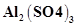(ii)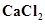(iii)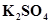(iv)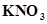(v)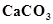Solution :

Question 9. What is meant by the term chemical formula?
Solution : A chemical formula is the representation of elements present in a compound with the help of symbols and also the number of atoms of each element with those numbers only. for eg: A molecule of water (compound) contains 2 atoms of hydrogen and one atom of oxygen hence its chemical formula is H2O.

Question 10. How many atoms are present in a
(i) H2S molecule and
(ii) PO43− ion?

Solution :
(i) In an H2S molecule, three atoms are present; two of hydrogen and one of sulphur.
(ii) In a PO43− ion, five atoms are present; one of phosphorus and four of oxygen.

Question 11. Calculate the molecular masses of H2, O2, Cl2, CO2, CH4, C2H6, C2H4, NH3, CH3OH.
Solution :
Molecular mass of H= 2 × Atomic mass of H
= 2 × 1
= 2 u
Molecular mass of O2 = 2 × Atomic mass of O
= 2 × 16
= 32 u
Molecular mass of Cl2 = 2 × Atomic mass of Cl
= 2 × 35.5
= 71 u
Molecular mass of CO2 = Atomic mass of C + 2 × Atomic mass of O
= 12 + 2 × 16
= 44 u
Molecular mass of CH4 = Atomic mass of C + 4 × Atomic mass of H
= 12 + 4 × 1
= 16 u
Molecular mass of C2H6 = 2 × Atomic mass of C + 6 × Atomic mass of H
= 2 × 12 + 6 × 1
= 30 u
Molecular mass of C2H4 = 2 × Atomic mass of C + 4 × Atomic mass of H
= 2 × 12 + 4 × 1
= 28 u
Molecular mass of NH= Atomic mass of N + 3 × Atomic mass of H
= 14 + 3 × 1
= 17 u
Molecular mass of CH3OH = Atomic mass of C + 4 × Atomic mass of H + Atomic mass of O
= 12 + 4 × 1 + 16
= 32 u

Question 12. Calculate the formula unit masses of ZnO, Na2O, K2CO3, given atomic masses of Zn = 65 u, Na = 23 u, K = 39 u, C = 12 u, and O = 16 u.
Solution :
Formula unit mass of ZnO = Atomic mass of Zn + Atomic mass of O
= 65 + 16
= 81 u
Formula unit mass of Na2O = 2 × Atomic mass of Na + Atomic mass of O
= 2 × 23 + 16
= 62 u
Formula unit mass of K2CO3 = 2 × Atomic mass of K + Atomic mass of C + 3 × Atomic mass of O
= 2 × 39 + 12 + 3 × 16
= 138 u

Question 13. If one mole of carbon atoms weighs 12 gram, what is the mass (in gram) of 1 atom of carbon?
Solution :
One mole of carbon atoms weighs 12 g (Given)
i.e., mass of 1 mole of carbon atoms = 12 g
Then, mass of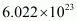number of carbon atoms = 12 g
Therefore, mass of 1 atom of carbon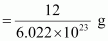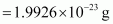Question 14. Calculate the formula unit masses of ZnO, Na2O, K2CO3, given atomic masses of Zn = 65 u, Na = 23 u, K = 39 u, C = 12 u, and O = 16 u.
Solution :
Formula unit mass of ZnO = Atomic mass of Zn + Atomic mass of O
= 65 + 16
= 81 u

Formula unit mass of Na2O = 2 × Atomic mass of Na + Atomic mass of O
= 2 × 23 + 16
= 62 u

Formula unit mass of K2CO3 = 2 × Atomic mass of K + Atomic mass of C + 3 × Atomic mass of O
= 2 × 39 + 12 + 3 × 16
= 138 u

Question 15. If one mole of carbon atoms weighs 12 gram, what is the mass (in gram) of 1 atom of carbon?
Solution :
One mole of carbon atoms weighs 12 g (Given)
i.e., mass of 1 mole of carbon atoms = 12 g
Then, mass ofnumber of carbon atoms = 12 g
Therefore, mass of 1 atom of carbonQuestion 16. Which has more number of atoms, 100 grams of sodium or 100 grams of iron (given, atomic mass of Na = 23 u, Fe = 56 u)?
Solution :
Atomic mass of Na = 23 u (Given)
Then, gram atomic mass of Na = 23 g
Now, 23 g of Na contains =number of atoms
Thus, 100 g of Na contains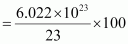number of atoms
=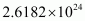number of atoms
Again, atomic mass of Fe = 56 u(Given)
Then, gram atomic mass of Fe = 56 g
Now, 56 g of Fe contains =number of atoms
Thus, 100 g of Fe contains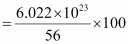number of atoms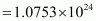number of atoms
Therefore, 100 grams of sodium contain more number of atoms than 100 grams of iron.

Question 17. A 0.24 g sample of compound of oxygen and boron was found by analysis to contain 0.096 g of boron and 0.144 g of oxygen. Calculate the percentage composition of the compound by weight.
Solution :

Mass of boron = 0.096 g (Given)
Mass of oxygen = 0.144 g (Given)
Mass of sample = 0.24 g (Given)
Thus, percentage of boron by weight in the compound =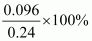= 40%
And, percentage of oxygen by weight in the compound =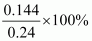= 60%

Question 18. When 3.0 g of carbon is burnt in 8.00 g oxygen, 11.00 g of carbon dioxide is produced. What mass of carbon dioxide will be formed when 3.00 g of carbon is burnt in 50.00 g of oxygen? Which law of chemical combination will govern your answer?
Solution : According to the law of chemical combination of constant proportions “in a chemical compound the elementary constituents always combine in constant proportions by weight/mass”. Therefore, whether 3 g carbon is burnt in 8 g oxygen or 3g carbon is burnt in 50g oxygen in both cases only 11g carbon dioxide will be formed.

Question 19. What are polyatomic ions? Give examples.
Solution :
A polyatomic ion is a group of atoms carrying a charge (positive or negative). For example, ammonium ion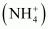, hydroxide ion (OH), carbonate ion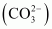, sulphate ion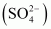.

Question 20. Write the chemical formulae of the following.
(a) Magnesium chloride
(b) Calcium oxide
(c) Copper nitrate
(d) Aluminium chloride
(e) Calcium carbonate.

Solution :

Question 21. Give the names of the elements present in the following compounds. (a) Quick lime
(b) Hydrogen bromide
(c) Baking powder
(d) Potassium sulphate.
Solution :

Question 22. Calculate the molar mass of the following substances: (a) Ethyne, C2H2
(b) Sulphur molecule, S8
(c) Phosphorus molecule, P(atomic mass of phosphorus = 31)
(d) Hydrochloric acid, HCl
(e) Nitric acid, HNO3
Solution :
(a) Molar mass of ethyne, C2H2 = 2 × 12 + 2 × 1 = 26 g
(b) Molar mass of sulphur molecule, S8 = 8 × 32 = 256 g
(c) Molar mass of phosphorus molecule, P4 = 4 × 31 = 124 g
(d) Molar mass of hydrochloric acid, HCl = 1 + 35.5 = 36.5 g
(e) Molar mass of nitric acid, HNO3 = 1 + 14 + 3 × 16 = 63 g

Question 23. What is the mass of−−
(a) 1 mole of nitrogen atoms?
(b) 4 moles of aluminium atoms (Atomic mass of aluminium = 27)?
(c) 10 moles of sodium sulphite (Na2SO3)?
Solution :
(a) The mass of 1 mole of nitrogen atoms is 14 g.
(b) The mass of 4 moles of aluminium atoms is (4 × 27) g = 108 g
(c) The mass of 10 moles of sodium sulphite (Na2SO3) is
10 × [2 × 23 + 32 + 3 × 16] g = 10 × 126 g = 1260 g

Question 24. Convert into mole.
(a) 12 g of oxygen gas
(b) 20 g of water
(c) 22 g of carbon dioxide.
Solution :
(a)molecular mass of O2= 32 u= 32g(1 mole)
since 32 g of O2=1mole then 12g of O2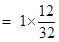=0.375mole.
(b)molecular mass of H2O+ 16 = 18 u= 18g(1mole)
20g H2O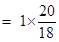= 1.11mole.
(c)molecular mass of CO2=12 + 16 x 2= 12 + 32 = 44 u= 44g (1mole)
22g of CO2=12 + 16 x 2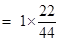= 0.5mole.

Question 25. What is the mass of :
(a) 0.2 mole of oxygen atoms?
(b) 0.5 mole of water molecules?
Solution :
(a) since 1 mole of O = atomic mass of O = 16u=16g
then 0.2mole of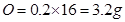(b)1mol of H2O = molecular mass of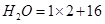=18 u =18g
then 0.5mol of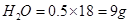Question 26. Calculate the number of molecules of sulphur (S8) present in 16 g of solid sulphur.
Solution :
1mol of S8= molecular mass of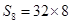= 256u=256g
since 256g of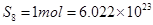atoms (Avogadro number)
16g of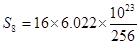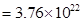molecules

Question 27. Calculate the number of aluminium ions present in 0.051 g of aluminium oxide.
(Hint: The mass of an ion is the same as that of an atom of the same element. Atomic mass of Al = 27 u)

Solution :
1mol of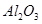= molecular mass of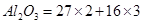= 102u=102g
aluminium ions present in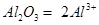102 g ofcontains aluminium ions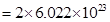then 0.051 gcontains aluminium ions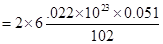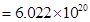Get 30% off your first purchase!

X
error: Content is protected !!
Scroll to Top